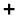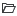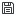# Calculate Data from Expressions

Use the Calculate tool to create new field quantities using a python expression. The new fields can be derived from existing fields or be completely independent of other results in the dataset.

There are some important characteristics of the Calculate tool that need to be understood to use it effectively. When a new function is created, it is not immediately evaluated. Rather, the expression syntax is parsed and checked for validity. If an expression contains invalid functions or variables it will not be accepted by the calculate context and the text will revert back to the previous state. Once the expression is validated, it is added to the global variable list within the session of HyperWorks CFD. It is not until that expression is selected to use for contouring, streamline tracing, or generating an iso-surface that it is evaluated. When the expression is evaluated, it is evaluated on a local basis. For example, you create an expression for total pressure:

pTot = 0.5*density*velocity**2 + pressure

If you then choose to color a slice plane by pTot, it is calculated based on the local coordinates and results variables available on that slice plane. No information from the parent volume dataset is accessed. This is done to minimize memory overhead of the derived variables and to improve performance. However, if your expression includes gradients of quantities, it is important to note that the gradients will not be calculated on a global level. Rather, they will be evaluated using the data on the surface. In the case of a slice plane, this means that the gradients will not be accurate in the plane normal direction. This “calculate on demand” approach also has some implications for querying the fields prior to use. A number of features in the user interface rely on the ability to query the function range. One example is the range setting of the colormap. For pre-existing variables, the range is populated based on a query of the range of the scalar. Since derived fields are not computed a priori, the range inputs will not be populated with proper values.

1. From the Post ribbon, click the Calculate tool.
The Derived Data Calculator dialog opens.
2. Clickto add a variable.
3. Define the name and expression.
• Define the name without spaces so that it can be utilized in further expression definitions.
• If using an existing variable with a space in its names, like “wall shear stress”, replace the spaces with “_”.
• Expressions are defined in python syntax.
Tip: Click/to import or export defined expressions.

The output of each expression produces new results at each node of the geometry that it is operating on. Results fields are stored as numpy arrays and can be operated on using standard numpy operations. For example, the x-component of the velocity array can be accessed with slicing by using the following syntax:

XVel = velocity[:,0]

Alternatively, the pre-defined field for the x component of the velocity could be directly referred to in an expression as follows:

XVelSquared = (x velocity)*(x velocity)

The expression shown above is not acceptable python syntax due to the space in the “x velocity” string. To overcome this, each expression is parsed prior to evaluation to convert the variable names to a valid python expression.

A number of internal functions are available for use in the Calculate context. The following table lists the variables and functions:
Function/Variable Description Example
Name Expression